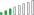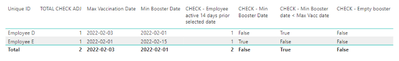cancel
Showing results for
Did you mean:Regular Visitor

## Calculating Total Number of Employees Using Max Date and Min Date Variable/Measure

I'm hoping that I can explain what I am needing and it makes sense.  I have 2 tables: a Calendar table that is used as a DAX generated table used for a date slicer and then an Employee table.

Below is a very small sample size of Employee data to help support what it is I am trying to do.
 Type Effective Date Manufacturer Unique ID Vaccination 05/19/2021 Unspecified Employee A Vaccination 02/03/2021 Moderna Employee B Booster 06/20/2022 Unspecified Employee B Vaccination 05/03/2022 Moderna Employee C Vaccination 02/03/2022 Moderna Employee D Booster 02/01/2022 Moderna Employee D Vaccination 02/01/2022 Moderna Employee E Booster 02/15/2022 Moderna Employee E

Within my report, I have a Date slicer that stores the selection of the end user within a measure shown below.

Selected Date = SELECTEDVALUE('Calendar'[Date])

Once a date is selected, I am needing to do the following:
1. Determine the Max Effective Date for each Unique ID when the employee has completed a Vaccination and the Effective Date of the Vaccination is less than or equal to 14 days prior to [Selected Date].  For example, if someone chose 4/1/22 as the [Selected Date], I would be looking for the Max [Effective Date] per [Unique ID] where [Type] = "Vaccination" and [Effective Date] <= 3/18/22 (14 days prior to 4/1/22).  For the next steps, let's refer to this Variable or Measure [Max Vaccination Effective Date].
2. Once [Max Vaccination Effective Date] is found, I need to find the Min Effective Date for each [Unique ID] where [Type] = "Booster" and [Effective Date] is greater than [Max Vaccination Effective Date].  For the next step, let's call this Variable or Measure [Min Booster Effective Date].
3. From there, I need to count the number of employees who completed a vaccination at least 14 days prior to the [Selected Date] and have either a [Min Booster Effective Date] that is before the employee's [Max Vaccination Effective Date], have a [Min Booster Effective Date] that is on or after the [Selected Date], or have not yet received a booster.

Using the sample table for Employees, I would expect the total number to be 3 (Employee A, Employee B, and Employee D).

I have built measures using all kinds of Filters, and the calculations are taking too much processing power and time to populate a result.  If anybody knows of the most simplistic way of getting these calculations, that would be great.  Please let me know if there is more context that I need to provide to help better illustrate what it is I am trying to do.

1 ACCEPTED SOLUTIONResolver III

Hi @joshua_dillon

Try to do something like this:
1. Disconnect the time table from your fact table.

2. Use this measure to check if the employee has an effective date between choosen date and choosen date minus 14 days:

CHECK - Employee active 14 days prior selected date = CALCULATE(DISTINCTCOUNT('Table'[Unique ID]),
FILTER( VALUES( 'Table'[Effective Date] ), 'Table'[Effective Date] <= MAX( 'Calendar'[Date] ) ),
FILTER( VALUES( 'Table'[Effective Date] ), OR( 'Table'[Effective Date] >= (MIN( 'Calendar'[Date] )-14), ISBLANK( 'Table'[Effective Date] ) ) ) )

3. Use this measure to find Maximum Vaccination Date:
Max Vaccination Date = calculate(MAX('Table'[Effective Date]),'Table'[Type]="Vaccination")

4. Use this measure to find the Minimum Booster Date that is after Maximum Vaccination Date:
Min Booster Date = CALCULATE(MIN('Table'[Effective Date]), FILTER('Table','Table'[Type]="Booster"&&'Table'[Effective Date]>[Max Vaccination Date]))

5. Use this measure to check the items listed in your 3rd point:

TOTAL CHECK = IF(
'Table'[CHECK - Employee active 14 days prior selected date]=1 &&
([Min Booster Date]<[Max Vaccination Date] || [Min Booster Date]>=MAX('Calendar'[Date]) || [Min Booster Date]=BLANK())
,1,0)

6. Use this measure to correct the counting of the sum in a matrix:
TOTAL CHECK ADJ = IF(HASONEFILTER('Table'[Effective Date]), 'Table'[TOTAL CHECK],SUMX('Table',[TOTAL CHECK]))

7. Then put the TOTAL CHECK ADJ measure in a visual level filter and set it to be equal or greater than 1.

For example, setting the date to 10.02.2022 I got these employees listed:Here you can find the test pbix file: https://filetransfer.io/data-package/NLwtgy9D#link
Please check if the solutions is ok.Resolver III

Hi @joshua_dillon

Try to do something like this:
1. Disconnect the time table from your fact table.

2. Use this measure to check if the employee has an effective date between choosen date and choosen date minus 14 days:

CHECK - Employee active 14 days prior selected date = CALCULATE(DISTINCTCOUNT('Table'[Unique ID]),
FILTER( VALUES( 'Table'[Effective Date] ), 'Table'[Effective Date] <= MAX( 'Calendar'[Date] ) ),
FILTER( VALUES( 'Table'[Effective Date] ), OR( 'Table'[Effective Date] >= (MIN( 'Calendar'[Date] )-14), ISBLANK( 'Table'[Effective Date] ) ) ) )

3. Use this measure to find Maximum Vaccination Date:
Max Vaccination Date = calculate(MAX('Table'[Effective Date]),'Table'[Type]="Vaccination")

4. Use this measure to find the Minimum Booster Date that is after Maximum Vaccination Date:
Min Booster Date = CALCULATE(MIN('Table'[Effective Date]), FILTER('Table','Table'[Type]="Booster"&&'Table'[Effective Date]>[Max Vaccination Date]))

5. Use this measure to check the items listed in your 3rd point:

TOTAL CHECK = IF(
'Table'[CHECK - Employee active 14 days prior selected date]=1 &&
([Min Booster Date]<[Max Vaccination Date] || [Min Booster Date]>=MAX('Calendar'[Date]) || [Min Booster Date]=BLANK())
,1,0)

6. Use this measure to correct the counting of the sum in a matrix:
TOTAL CHECK ADJ = IF(HASONEFILTER('Table'[Effective Date]), 'Table'[TOTAL CHECK],SUMX('Table',[TOTAL CHECK]))

7. Then put the TOTAL CHECK ADJ measure in a visual level filter and set it to be equal or greater than 1.

For example, setting the date to 10.02.2022 I got these employees listed:Here you can find the test pbix file: https://filetransfer.io/data-package/NLwtgy9D#link
Please check if the solutions is ok.